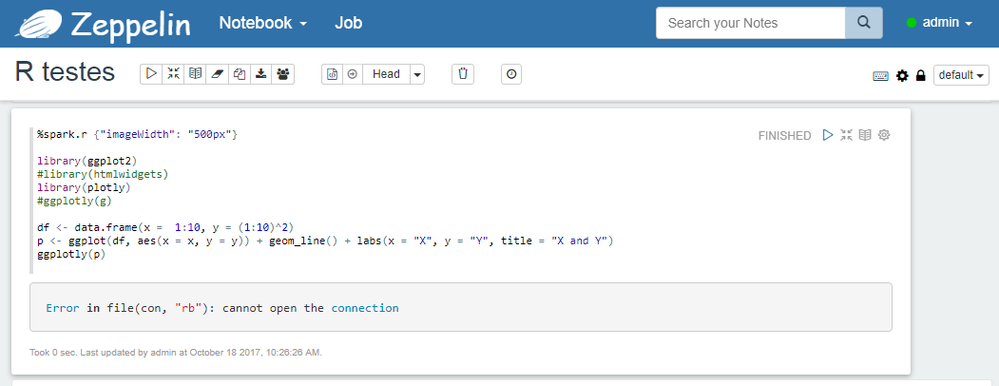Support Questions
Announcements
Alert: Welcome to the Unified Cloudera Community. Former HCC members be sure to read and learn how to activate your account here.

## Unable to use R plotly on Zeppelinsaulo_sobreiro
Explorer

Hi everyone,

I am having an issue running the plotly library on zeppelin using %spark.r interpreter. The command output is always:
"Error in file(con, "rb"): cannot open the connection"
as you can see in the attached image.

If it is not possible to see the image, the notebook code looks like the following:

```%spark.r {"imageWidth": "500px"}

library(ggplot2)
#library(htmlwidgets)
library(plotly)

df <- data.frame(x =  1:10, y = (1:10)^2)
p <- ggplot(df, aes(x = x, y = y)) + geom_line() + labs(x = "X", y = "Y", title = "X and Y")
ggplotly(p)```

I am using:

• HDP 2.6.2.0-205
• Zeppelin 0.7.2
• Spark 1.6.3
• R 3.4.1

ggplot2 is working properly.

Can someone tell me how can I solve this problem?